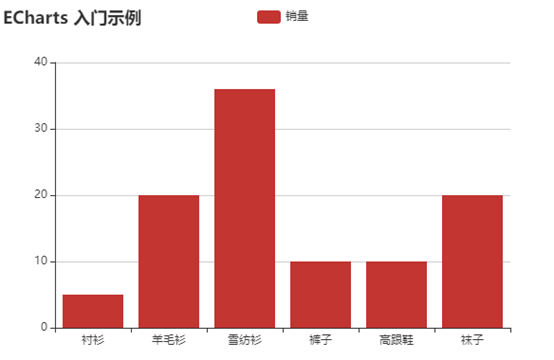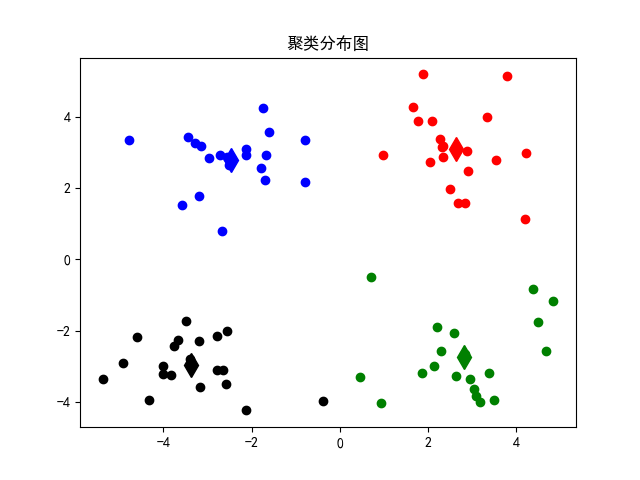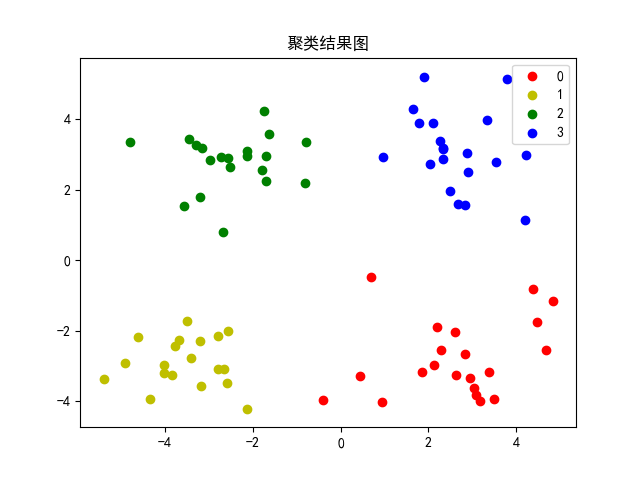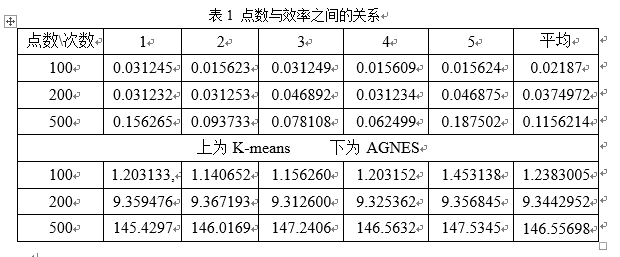•文档
• 任选一支股票或大盘指数的日收益率数据（观测值不少于1000个），观察数据分布特点，计算其VaR（Value at Risk）及CVaR（Conditional VaR），可以考虑运用各种方法计算并进行比较。
• Eviews计算CoVaR的步骤，分位数回归方法和GARCH方法。
• 主线程在循环中不断地对shared_var 进行加1操作，即每次循环shared_var 被加1；而新创建的线程则不断地对shared_var 进行减1 操作，即每次循环shared_var 被减1。观察程序运行的结果，并对你看到的现象进行解释。
• 1．实现文档对象的属性； 2．实现文档对象的属性方法； 3．... ...答： Var tables = document.getElementsByTagName("table"); alert("This document contains contains"+tables.length+"tables");
• 操作系统上机实验报告 实验名称 进程和线程 实验目的 理解uix/Linux下进程和线程的创建并发执行过程 实验内容 1进程的创建 2多线程应用 实验步骤及分析 进程的创建 下面这个C程序展示了UNIX系统中父进程创建子进程及...
• 实验室名称： 指导老师： 实训日期： 年 月 日 实训题目：Echarts数据可视化 实训目的： 一、软件Hbuilder 的安装与基本使用 二、创建项目：HTML文件、JS文件、CSS文件基本使用 三、新建项目、Web可视化项目 输入...
课程：数据可视化 实验室名称： 指导老师： 实训日期： 年 月 日 实训题目：Echarts数据可视化 实训目的： 一、软件Hbuilder 的安装与基本使用 二、创建项目：HTML文件、JS文件、CSS文件基本使用 三、新建项目、Web可视化项目 输入项目名称后继续 四、Echarts资源下载 五、Echarts官方网站运行10个官方案例 六、在Echarts官方网站查看快速入门文档 七、自行编写数据源 实现案例 折线图 柱状图 饼状图 实训内容：
<!DOCTYPE html>
<html>
<meta charset="utf-8" />
<title>ECharts</title>
<!-- 引入刚刚下载的 ECharts 文件 -->
<script src="echarts.min.js"></script>
<body>
<!-- 为 ECharts 准备一个定义了宽高的 DOM -->
<div id="main" style="width: 600px;height:400px;"></div>
<script type="text/javascript">
// 基于准备好的dom，初始化echarts实例
var myChart = echarts.init(document.getElementById('main'));

// 指定图表的配置项和数据
var option = {
title: {
text: 'ECharts 入门示例'
},
tooltip: {},
legend: {
data: ['销量']
},
xAxis: {
data: ['衬衫', '羊毛衫', '雪纺衫', '裤子', '高跟鞋', '袜子']
},
yAxis: {},
series: [
{
name: '销量',
type: 'bar',
data: [5, 20, 36, 10, 10, 20]
}
]
};

// 使用刚指定的配置项和数据显示图表。
myChart.setOption(option);
</script>
</body>
</html>

option = {
xAxis: {
type: 'category',
data: ['Mon', 'Tue', 'Wed', 'Thu', 'Fri', 'Sat', 'Sun']
},
yAxis: {
type: 'value'
},
series: [
{
data: [120, 200, 150, 80, 70, 110, 130],
type: 'bar',
showBackground: true,
backgroundStyle: {
color: 'rgba(180, 180, 180, 0.2)'
}
}
]
};

页面效果：展开全文数据可视化 Echarts
•  熟悉linux环境的基本使用命令，熟悉使用vi、gdb、gcc等编程工具或软件  利用fork()函数创建子进程。  考察fork()函数创建的子进程与父进程之间的同名变量是否为临界资源...实验报告（含流程图及运行结果）&源码进程
• LINUX 操作系统实验报告;换与别名管道及输入输出重定向 实验内容 1使用shutdown命令设定在30分钟之后关闭计算机 2使用命令cat /etc/cron.daliy设置为别名named然后再取消别名 3使用echo命令和输出重定向创建文本文件...
• ## js实验报告

千次阅读 2020-09-11 11:32:24
【js实验一】 1.编写一个JavaScript程序，实现在页面上输出“欢迎访问我的个人主页”，效果如图所示 2.编写一个JavaScript程序，效果如图所示。 3.编写一个JavaScript程序，效果如图所示。 4.按要求实现如下界面： ...
【js实验一、二】 1.编写一个JavaScript程序，实现在页面上输出“欢迎访问我的个人主页”，效果如图所示
<body>
<script type="text/javascript">
document.write("<hr />");
document.write("<h1>欢迎访问我的个人主页</h1>")
document.write("<hr />");
</script>
</body>

2.编写一个JavaScript程序，效果如图所示。<body>
<script type="text/javascript">
var name = prompt("What's your name?");
var demanding = "can";
var tech = "dance";
var techy = name+" "+demanding+" "+tech;
document.writeln(techy);
</script>
</body>

3.编写一个JavaScript程序，效果如图所示。<body>
<script type="text/javascript">
var name = prompt("你的名字是？");
var demanding = "会";
var tech = "唱歌。";
var techy = name+demanding+tech;
document.writeln(techy);
document.writeln(techy.bold())
document.writeln(techy.fontcolor('red'));
</script>

</body>

4.按要求实现如下界面：其中var a=1;var b=2; var c=3;（alert弹出对话框）
<body>
<script type="text/javascript">
var a = 1;
var b = 2;
var c = 3;
</script>
</body>

5.<body>
<pre>
<script type="text/javascript">
var a = 2009;
var b = "相信明天会更好",result;
var c = true;
document.writeln("a=2009,b='相信明天会更好'，c=true");
document.writeln("");
document.write("a+b=");result=a+b;document.writeln(result);
document.write("b+a=");result=b+a;document.writeln(result);
document.write("b+c=");result=b+c;document.writeln(result);
document.write("c+b=");result=c+b;document.writeln(result);
document.write("a+b+c=");result=a+b+c;document.writeln(result);
</script>
</pre>


按要求实现如下界面：其中a=1 b=2
<body>
<script type="text/javascript">
var a = 1;
var b = 2;
var c = 0;
document.writeln("a=1,b=2<br />");
document.write("a+=b = ");result=a+=b; document.writeln(result);
document.write("b+=a = ");result=b+=a; document.writeln(result);
document.write("a-=b = ");result=a-=b; document.writeln(result);
document.write("b*=a = ");result=b*=a; document.writeln(result);
</script>
</body>

7.设计一个程序，小高今年22岁，应用条件判断语句，大于18岁就是成年人，否则为未成年人，并输出结果。
<body>
<script type="text/javascript">
var age = 22;
if (age>=18) {
} else{
}
</script>
</body>

8.按要求实现如下界面：（alert弹出对话框）其中a=1+2*3， b=(1+2)*3
<body>
<script type="text/javascript">
var a = 1+2*3;
var b = (1+2)*3;

</script>
</body>

9.设计一个程序，返回true的数据类型。
<body>
<script type="text/javascript">
var a = true;
document.writeln(typeof(a));
</script>

</body>

10.输入一个四位年份，判断是否为闰年
<body>
<script type="text/javascript">
var year = prompt("请输入一个四位年份");
if (year%4==0&year%100!=0||year%400==0) {
} else{
}
</script>
</body>

展开全文js
• VAR模型及蒙特卡罗模拟（张晓彤），可以用于风险评估，适合数学建模时使用，配合Eviews软件
• 在大数据领域这个聚类算法真是起到了十分重要的作用，...具体的实验报告和python源码文件在最后位置，提供百度云下载，本文使用的是K-means算法和层次聚类算法AGNES，原理介绍和实验结果详见百度云提供的报告等 ...
在大数据领域这个聚类算法真是起到了十分重要的作用，只有通过有效地聚类才能得到非常直观的结果。
有一个实验要求对比两种大数据聚类算法的性能，具体的代码也不是由我实现的，我只是改了一部分，主要还是博客大佬们的代码，我这里借用了一下~~ 具体的实验报告和python源码文件在最后位置，提供百度云下载，本文使用的是K-means算法和层次聚类算法AGNES，原理介绍和实验结果详见百度云提供的报告等
如今大数据的时代，大量的可以获得的数据被保存，用来分析以获得有用的信息，然而数据纷繁杂乱，需要研究者对齐进行一定的划分，将相似的数据放在一起进行分析，这就是所谓的聚类。
聚类就是按照某个特定标准(如距离准则，即数据点之间的距离)把一个数据集分割成不同的类或簇，使得同一个簇内的数据对象的相似性尽可能大，同时不在同一个簇中的数据对象的差异性也尽可能地大，常用的基本的距离计算方式就是欧氏距离。我们可以具体地理解为，聚类后同一类的数据尽可能聚集到一起，不同类数据尽量分离。聚类技术正在蓬勃发展，应用到的领域包括数据挖掘、统计学、机器学习、空间数据库技术、生物学以及市场营销等。各种聚类方法也被不断提出和改进，而不同的方法适合于不同类型的数据，因此对各种聚类方法、聚类效果的比较是一个值得研究的问题。
目前，有大量的聚类算法。而对于具体应用，聚类算法的选择取决于数据的类型、聚类的目的。如果聚类分析被用作描述或探查的工具，可以对同样的数据尝试多种算法，以发现数据可能揭示的结果。主要的聚类算法可以划分为如下几类：划分方法、层次方法、基于密度的方法、基于网格的方法以及基于模型的方法。
K-means聚类算法
K-means是划分方法中较经典的聚类算法之一。由于该算法的效率高，所以在对大规模数据进行聚类时被广泛应用。目前，许多算法均围绕着该算法进行扩展和改进。K-means算法目标是，以k为参数，把n个对象分成k个簇，使簇内具有较高的相似度，而簇间的相似度较低。 K-means算法的处理过程如下：首先，随机地 选择k个对象，每个对象初始地代表了一个簇的平均值或中心;对剩余的每个对象，根据其与各簇中心的距离，将它赋给最近的簇;然后重新计算每个簇的平均值。 这个过程不断重复，直到准则函数收敛。 通常，采用平方误差准则。平方误差准则采用的是数据库中所有对象的平方误差的总和，与是空间中的点和每个簇的的平均值有关。目标是使生成的簇尽可能紧凑独立，使用的距离度量是欧几里得距离,当然也可以用其他距离度量。K-means聚类算法的算法流程如下： 输入：包含n个对象的数据库和簇的数目k； 输出：k个簇，使平方误差准则最小。 步骤： (1) 任意选择k个对象作为初始的簇中心； (2) repeat； (3) 根据簇中对象的平均值，将每个对象(重新)赋予最类似的簇； (4) 更新簇的平均值，即计算每个簇中对象的平均值； (5) until不再发生变化。 优点：简单直接（体现在逻辑思路以及实现难度上），易于理解，在低维数据集上有不错的效果（简单的算法不见得就不能得到优秀的效果）。 缺点：对于高维数据（如成百上千维，现实中还不止这么多），其计算速度十分慢，主要是慢在计算距离上（参考欧几里得距离，当然并行化处理是可以的，这是算法实现层面的问题），它的另外一个缺点就是它需要我们设定希望得到的聚类数k，若我们对于数据没有很好的理解，那么设置k值就成了一种估计性的工作，并且会对实验结果造成很大的偏差。
层次聚类算法
根据层次分解的顺序是自底向上的还是自上向下的，层次聚类算法分为凝聚的层次聚类算法和分裂的层次聚类算法。 凝聚型层次聚类的策略是先将每个对象作为一个簇，然后合并这些原子簇为越来越大的簇，直到所有对象都在一个簇中，或者某个终结条件被满足。绝大多数层次聚类属于凝聚型层次聚类，它们只是在簇间相似度的定义上有所不同。四种广泛采用的簇间距离度量方法为：最小距离，最大距离，平均值距离，平均距离。 这里以最小距离的凝聚层次聚类算法流程为例： (1) 将每个对象看作一类，计算两两之间的最小距离； (2) 将距离最小的两个类合并成一个新类； (3) 重新计算新类与所有类之间的距离； (4) 重复(2)、(3)，直到所有类最后合并成一类。 优点：
距离和规则的相似度容易定义，限制少；不需要预先制定聚类数；可以发现类的层次关系（在一些特定领域如生物有很大作用）； 缺点：计算复杂度太高（考虑并行化）；奇异值也能产生很大影响；算法很可能聚类成链状（一层包含着一层）；算法不需要预定聚类数，但是我们选择哪个层次的聚类作为我们需要的聚类效果，这需要我们按照实际客观情况以及经验来完成，毕竟就凝聚聚类来说，从最底层的每个个体作为一个个体，到最顶层所有个体合并为一个个体，其中的聚类结果可能有许许多多种。当然针对这个问题也有许多解决方案，其中一个常用的就是凝聚到某个程度其聚类之间的距离都大于某个阈值k，就停止凝聚。
具体代码
在实验中，本人先是下载的点集，然后为了测试效率写了一个简单的产生大量点的脚本，具体的实现代码如下（来自网络 进行了小小的更改）,注意需要的库文件，没有的安装一下，还有路径也需要改： AGNES
#-*- coding:utf-8 -*-
import math
import pylab as pl
import codecs
import re
import datetime

pl.rcParams['axes.unicode_minus'] = False
# 为了显示汉字坐标的

#计算欧式距离,a,b代表两个元组
def calcudistance(a, b):
return math.sqrt(math.pow(a-b, 2)+math.pow(a-b, 2))

# 求出最小距离
def dist_min(Ci, Cj):
return min(calcudistance(i, j) for i in Ci for j in Cj)

# 求出最大距离
def dist_max(Ci, Cj):
return max(calcudistance(i, j) for i in Ci for j in Cj)

#求出平均距离
def dist_avg(Ci, Cj):
return sum(calcudistance(i, j) for i in Ci for j in Cj)/(len(Ci)*len(Cj))

#找到距离最小的下标
def find_Min(M):
min = 1000
x = 0; y = 0
for i in range(len(M)):
for j in range(len(M[i])):
if i != j and M[i][j] < min:
min = M[i][j];x = i; y = j
return (x, y, min)

#算法核心
def AGNES(dataset, dist, k):

#初始化C和M
C = [];M = []
for i in dataset:
Ci = []
Ci.append(i)
C.append(Ci)
for i in C:
Mi = []
for j in C:
Mi.append(dist(i, j))
M.append(Mi)
q = len(dataset)
#合并更新
while q > k:
x, y, min = find_Min(M)
C[x].extend(C[y])
C.remove(C[y])
M = []
for i in C:
Mi = []
for j in C:
Mi.append(dist(i, j))
M.append(Mi)
q -= 1
return C

# 画出结果图
def drawfig(C):
colValue = ['r', 'y', 'g', 'b', 'c', 'k', 'm']  # 颜色数组
for i in range(len(C)):
coo_X = []    # x坐标
coo_Y = []    # y坐标
for j in range(len(C[i])):
coo_X.append(C[i][j])
coo_Y.append(C[i][j])
pl.scatter(coo_X, coo_Y, marker='o', color=colValue[i%len(colValue)], label=i)

pl.legend(loc='upper right')
pl.title("聚类结果图")
pl.savefig(savepath + '2.png')
pl.show()

def draworigian(dataset):
x_list = list()
y_list = list()
for i in range(len(dataSet)):
temp = dataSet[i]
x_list.append(temp)
y_list.append(temp)
pl.scatter(x_list, y_list, marker='o', color="b")
pl.legend(loc='upper right')
pl.title("数据原始分布")
pl.savefig(savepath + '1.png')
pl.show()

# 读取文本 保存为二维点集
dataSet = list()
for line in inDate:  # 段落的处理
line = line.strip()
strList = re.split('[ ]+', line)
numList = list()
for item in strList:
num = float(item)
numList.append(num)
# print numList
dataSet.append(numList)
return dataSet  # dataSet = [[], [], [], ...]

savepath='D:/学习/研1/模式识别/AGNES/'
Filepath = "D:/学习/研1/模式识别/testSet.txt"  # 数据集文件
draworigian(dataSet)

start = datetime.datetime.now()
result = AGNES(dataSet, dist_avg, 4)
end = datetime.datetime.now()
timeused = end - start
print(timeused)

drawfig(result)

# 100   1.203133, 01.140652, 1.156260, 1.203152, 1.453138
# 200点 9.359476, 09.367193, 09.312600, 09.325362, 09.356845
# 500点 147.946446, 147:351248, 147.153595,147.946446, 145.493638

# 500 无需 145.429797 146.016936  147.240645  146.563253 147.534587

k-MEANS
# -*- coding: UTF-8 -*-
import numpy
import random
import codecs
import re
import matplotlib.pyplot as plt
import datetime
plt.rcParams['axes.unicode_minus'] = False  # 显示负号

# 计算欧式距离,a,b代表两个向量
def calcudistance(a,b):
return numpy.sqrt(numpy.sum(numpy.square(a - b)))

# 初始化k个质心，数据集中随机选择
def initcentroids(dataSet, k):
return random.sample(dataSet, k)  # 从dataSet中随机获取k个数据项返回

def mindistance(dataSet, centroidList):
# 对每个属于dataSet的item，计算item与centroidList中k个质心的欧式距离，找出距离最小的，
# 并将item加入相应的簇类中

clusterDict = dict()  # 用dict来保存簇类结果
for item in dataSet:
vec1 = numpy.array(item)  # 转换成array形式
flag = 0  # 簇分类标记，记录与相应簇距离最近的那个簇
minDis = float("inf")  # 初始化为最大值

for i in range(len(centroidList)):
vec2 = numpy.array(centroidList[i])
distance = calcudistance(vec1, vec2)  # 计算相应的欧式距离
if distance < minDis:
minDis = distance
flag = i  # 循环结束时，flag保存的是与当前item距离最近的那个簇标记

if flag not in clusterDict.keys():  # 簇标记不存在，进行初始化
clusterDict[flag] = list()
# print flag, item
clusterDict[flag].append(item)  # 加入相应的类别中

return clusterDict  # 返回新的聚类结果

def getCentroids(clusterDict):
# 得到k个质心
centroidList = list()
for key in clusterDict.keys():
centroid = numpy.mean(numpy.array(clusterDict[key]), axis=0)  # 计算每列的均值，即找到质心
# print key, centroid
centroidList.append(centroid)

return numpy.array(centroidList).tolist()

def getVar(clusterDict, centroidList):
# 计算簇集合间的均方误差
# 将簇类中各个向量与质心的距离进行累加求和

sum = 0.0
for key in clusterDict.keys():
vec1 = numpy.array(centroidList[key])
distance = 0.0
for item in clusterDict[key]:
vec2 = numpy.array(item)
distance += calcudistance(vec1, vec2)
sum += distance

return sum

# 画出结果他图
def drawfig(centroidList, clusterDict):
# 展示聚类结果
global imgcount
imgcount += 1
colorMark = ['or', 'ob', 'og', 'ok', 'oy', 'ow']  # 不同簇类的标记 'or' --> 'o'代表圆，'r'代表red，'b':blue
centroidMark = ['dr', 'db', 'dg', 'dk', 'dy', 'dw']  # 质心标记 同上'd'代表棱形
for key in clusterDict.keys():
plt.plot(centroidList[key], centroidList[key], centroidMark[key], markersize=12)  # 画质心点
for item in clusterDict[key]:
plt.plot(item, item, colorMark[key])  # 画簇类下的点

plt.title("聚类分布图")
plt.savefig(savepath + str(imgcount) + '.png')
plt.show()

# 关键部分
def k_means(dataset,kindnum):
centroidList = initcentroids(dataSet, 4)  # 初始化质心，设置k=4
clusterDict = mindistance(dataSet, centroidList)  # 第一次聚类迭代
newVar = getVar(clusterDict, centroidList)  # 获得均方误差值，通过新旧均方误差来获得迭代终止条件
oldVar = -0.0001  # 旧均方误差值初始化为-1
print("----- 第1次迭代 -----")
print('k个均值向量: ')
print(centroidList)
print('平均均方误差: %d' % (newVar))
# drawfig(centroidList, clusterDict)  # 展示聚类结果
k = 2
while abs(newVar - oldVar) >= 0.0001:  # 当连续两次聚类结果小于0.0001时，迭代结束
centroidList = getCentroids(clusterDict)  # 获得新的质心
clusterDict = mindistance(dataSet, centroidList)  # 新的聚类结果
oldVar = newVar
newVar = getVar(clusterDict, centroidList)
print('----- 第%d次迭代 -----\n簇类' %(k))
print('k个均值向量: ')
print(centroidList)
print('平均均方误差: %d' %(newVar))
k += 1  # 迭代次数
return (centroidList,clusterDict)

# 读取文本 保存为二维点集
dataSet = list()
for line in inDate:
line = line.strip()
strList = re.split('[ ]+', line)  # 去除多余的空格
# print strList, strList
numList = list()
for item in strList:
num = float(item)
numList.append(num)
# print numList
dataSet.append(numList)
return dataSet  # dataSet = [[], [], [], ...]

savepath = 'D:/学习/研1/模式识别/K-means/'
imgcount = 0
Filepath = "D:/学习/研1/模式识别/testSet.txt"  # 数据集文件

start = datetime.datetime.now()
centroidList, clusterDict = k_means(dataSet, 4)
end = datetime.datetime.now()
timeused = end - start
print(timeused)

drawfig(centroidList, clusterDict)  # 展示聚类结果

# 100   0.031245, 0.015623, 0.031249, 0.015609, 0.015624
# 200点 0.031232, 0.031253, 0.046892, 0.031234, 0.046875
# 500点 0.156265, 0.093733, 0.078108,0.062499, 0.187502
# 10000 2.000017

# 无顺序 500 00.218750 00.547491 00.421866 00.281266 00.281266

最后这是是一个产生点集的脚本，分两种，一个是无序的随机，另一个是大概扎堆的，一个是为了检测效率，一个是为了测试效果。
import numpy as np
import copy

choosetype = 2  # 1 表示有序点 其他表示随机点
data = [[3.5, -3.5], [3.5, 3.5], [-3.5, 3.5], [-3.5, -3.5]]  # 四类点的中心
totalnum =500    #产生点的个数
file_path = "D:\学习\研1\模式识别\\testSet2.txt"  # 保存路径

fopen = open(file_path, 'w')  # 追加的方式读写打开

for i in range(totalnum):
if choosetype == 1:
datatemp = copy.deepcopy(data)
choose = datatemp[i % 4]
n1 = 2*np.random.random(1) - 1
n2 = 2*np.random.random(1) - 1
choose = choose + n1
choose = choose + n2
fopen.writelines(str(round(choose, 6)) + "   " + str(round(choose, 6)) + "\n")
else:
n1 = 4 * np.random.random(1) - 2
n2 = 4 * np.random.random(1) - 2

fopen.writelines(str(round(n1, 6)) + "   " + str(round(n2, 6)) + "\n")
fopen.close()

实验结果k-means测试结果，该算法有效AGNES的结果，算法有效
为了比较性能，对大量的点进行实验，表格如下：具体的算法原理和实验分析见报告内容，最后面提供百度云源码和报告的连接
心得体会
大数据可以说是现在研究十分火热的一个课题，在众多的研究室和科技公司等都是属于一个较为核心的项目。尤其是在当今网络迅速发展的时代，信息就意味着资源，如果能掌握信息就能把握机会。作为大数据分析的关键环节，聚类为分析者提供了效果超凡的数据预处理，使得我们可以发现在数据之下隐藏的逻辑关系与形势。
在众多的聚类算法之中，我们对两个基本的算法K-means和AGNES进行了了解和学习，并实用python语言来实现和对比两者的效果。结果表明，从大数据量的运算来讲，K-means快很多，但AGNES对数据的适用性更高。
在模式识别课程上，学习到了很多有关的人工智能和大数据分析等的知识，可以说获益匪浅。经过动手做实验，更加加深了我对聚类方法的理解，有助于以后更加深入的学习。首先，我要感谢老师耐心细致的授课，对基本概念的讲解十分简明易懂，采用的方式也让人容易理解。其次，感谢我的组员同学们对我实验期间的帮助，共同进步，共同提高。
下载地址： 链接: https://pan.baidu.com/s/1r0MXJSKO5uKq4hWa5IL0Dw 密码: 6sd3
展开全文大数据 聚类算法 python编程 k-means
• 实验一 词法分析 1．实验目的 （1）根据 PL/0 语言的文法规范，编写PL/0语言的词法分析程序；或者调研词法分析程序的自动生成工具LEX或FLEX，设计并实现一个能够输出单词序列的词法分析器。 （2） 通过设计调试词法...
实验一 词法分析 1．实验目的 （1）根据 PL/0 语言的文法规范，编写PL/0语言的词法分析程序；或者调研词法分析程序的自动生成工具LEX或FLEX，设计并实现一个能够输出单词序列的词法分析器。 （2） 通过设计调试词法分析程序，实现从源程序中分出各种单方法；加深对课堂教学的理解；提高词法分析方法的实践能力。 （3）掌握从源程序文件中读取有效字符的方法和产生源程序的内部表示文件的法。 （4）掌握词法分析的实现方法。 （5）上机调试编出的词法分析程序。 2．实验内容 已给 PL/0 语言文法，输出单词符号（关键字、专用符号以及其它标记）。 把词法分析器设计成一个独立一遍的过程。 词法分析器的输出形式采用二元式序列，即： (单词种类, 单词的值)。
3．实验步骤 （1）写出各个表达式的正则式 关键字的正则式
标识符的正则式
常数的正则式
运算符的正则式
界符的正则式
（2）根据正则式画出NFA
利用以上三条规则对r进行分裂：
（3）根据确定化的NFA画出DFA,并对DFA化简
根据化简规则发现B，D和C，E可以合并 简化后的DFA为：
（4）画出程序的算法流程图
（5）编写代码并测试结果 代码放在附录中（实验总结之后）
4.实验总结 （1）通过先写出正则式到NFA到DFA再到画出程序的流程图，一步一步的优化自己的编程流程，可以在自己脑海中形成清晰的框架，不会出现一些大的方向上的判断错误。 （2）在程序编写时，用到了C++自带的库函数strtok，可以将字符串按照给定的多个一位分割符进行分割，将界符，运算符和其他区分开，便于遍历。在调试程序过程中，一开始在多位运算符号的判定出现问题，字符串数组的下标的值未能及时修改。之后调用修改下标函数专门对下标进行修改。 （3）通过这次实验理解了从正则式到最简DFA的每一步的含义。正规式是正规集的表现形式，通过正规式更好的人工设计成NFA（NFA与正规式的等价性），根据NFA运用子集法转换成DFA，解决了更容易编程问题（DFA和NFA的等价性），最后用最小化简法对DFA进行化简，以达到最简的效果，有利于编程实现。 （4）通过这次实验对于之前学到的词法分析有了进一步的了解，加深了对于词法分析的步骤的理解与领悟。对于今后对编译原理的学习有很大的帮助。 5.附录（实验代码） #include <bits/stdc++.h> //万能头文件 using namespace std; string input; //输入全局 int cnt; //全局变量 bool judge (string input, string s) ///判断是否和目标串匹配 { int i=0; if (input.length()!=s.length()) return false; else { for(i=0;i<s.length();i++) { if(input[i]!=s[i]) return false; ///遍历 } return true; } } void feifuhao(string p) ///判断非符号的程序，包含判断关键字，标识符，常数 { if(judge (p,“begin”)) ///判断是否跟目标串相同，相同的话输出结果 { cout<<"("; cout<<“beginsym,”; cout<<p; cout<<")\n"; } else if(judge (p,“call”)) ///判断是否跟目标串相同，相同的话输出结果 { cout<<"("; cout<<“callsym,”; cout<<p; cout<<")\n"; } else if(judge (p,“const”)) ///判断是否跟目标串相同，相同的话输出结果 { cout<<"("; cout<<“constsym,”; cout<<p; cout<<")\n"; } else if(judge (p,“do”)) ///判断是否跟目标串相同，相同的话输出结果 { cout<<"("; cout<<“dosym,”; cout<<p; cout<<")\n"; } else if(judge (p,“end”)) ///判断是否跟目标串相同，相同的话输出结果 { cout<<"("; cout<<“endsym,”; cout<<p; cout<<")\n"; } else if(judge (p,“if”)) ///判断是否跟目标串相同，相同的话输出结果 { cout<<"("; cout<<“ifsym,”; cout<<p; cout<<")\n"; } else if(judge (p,“odd”)) ///判断是否跟目标串相同，相同的话输出结果 { cout<<"("; cout<<“oddsym,”; cout<<p; cout<<")\n"; } else if(judge (p,“procedure”)) ///判断是否跟目标串相同，相同的话输出结果 { cout<<"("; cout<<“proceduresym,”;cout<<p; cout<<")\n"; } else if(judge (p,“read”)) ///判断是否跟目标串相同，相同的话输出结果 { cout<<"("; cout<<“readsym,”;cout<<p; cout<<")\n"; } else if(judge (p,“var”)) ///判断是否跟目标串相同，相同的话输出结果 { cout<<"("; cout<<“varsym,”;cout<<p; cout<<")\n"; } else if(judge (p,“then”)) ///判断是否跟目标串相同，相同的话输出结果 { cout<<"("; cout<<“thensym,”;cout<<p; cout<<")\n"; } else if(judge (p,“write”)) ///判断是否跟目标串相同，相同的话输出结果 { cout<<"("; cout<<“writesym,”;cout<<p; cout<<")\n"; } else if(judge (p,“while”)) ///判断是否跟目标串相同，相同的话输出结果 { cout<<"("; cout<<“whilesym,”;cout<<p; cout<<")\n"; } else { int flag=0; for(int i=0;i<p.length();i++) { if(!isdigit(p[i])) //判断是否是标识符 { flag=1; cout<<"("; cout<<“ident,”; cout<<p; cout<<")\n"; break; } } if(!flag) //判断是否是数字 { cout<<"("; cout<<“number,”; cout<<p; cout<<")\n"; } } } int change(string aa,int cnt) ///防止多个运算符组成，返回正确下标 { int x=0; char f={’+’,’-’,’*’,’/’,’=’,’<’,’>’,’:’,’(’,’)’,’,’,’;’,’.’}; for(int i=0;i<13;i++) { if(aa[cnt]f[i]) { x=i; } } if(x5) { if(aa[cnt+1]’>’) //如果运算符是两个符号组成，cnt+1 { cnt=cnt+1; return cnt; } else if(aa[cnt+1]’=’) //判断两个运算符相连 { cnt=cnt+1; return cnt; } } if(x==7) //判断：= { cnt=cnt+1; return cnt;
}
return cnt;

} void fuhao (string aa,int cnt) ///对运算符和界符的输出 { int x=0; char f={’+’,’-’,’’,’/’,’=’,’<’,’>’,’:’,’(’,’)’,’,’,’;’,’.’}; for(int i=0;i<13;i++) { if(aa[cnt]f[i]) { x=i; } } if(x0) cout<<"(plus,+)"<<endl; if(x1) cout<<"(minus,-)"<<endl; if(x2) cout<<"(times,)"<<endl; if(x3) cout<<"(slash,/)"<<endl; if(x4) cout<<"(eql,=)"<<endl; if(x5) { if(aa[cnt+1]’>’) { cnt=cnt+1; cout<<"(neq,<>)"<<endl; } else if(aa[cnt+1]’=’) { cout<<"(leq,<=)"<<endl; } else { cout<<"(lss,<)"<<endl; } } if(x6) { if(aa[cnt+1]’=’) { cout<<"(geq,>=)"<<endl; } else { cout<<"(gtr,>)"<<endl; } } if(x7) { cout<<"(becomes,:=)"<<endl; } if(x8) cout<<"(lparen,()"<<endl; if(x9) cout<<"(rparen,))"<<endl; if(x10) cout<<"(comma,)"<<endl; if(x11) cout<<"(semicolon,😉"<<endl; if(x==12) cout<<"(period,.)"<<endl; } int main() { string aa; while(cin>>aa) { cnt=0; const char d = " ±/=<>:(),;."; char p; ///运用空格和运算符和界符分割字符串并且遍历 char buf ; strcpy(buf , aa.c_str()); //字符串转成数组 p = strtok(buf,d); //p是一个char while§ { if(aa[cnt]==p) //当前无符号 { feifuhao§; cnt=cnt+strlen§; } else ///当前是符号 { fuhao(aa,cnt); cnt=change(aa,cnt); cnt=cnt+1; feifuhao§; cnt=cnt+strlen§; } p=strtok(NULL,d); //下移一位，进行遍历 } for(int i=cnt;i<aa.length();i++) { //cout<<aa[i]; fuhao(aa,i); //防止最后有多个符号 } } return 0;}
展开全文• } catch (e) { console.log("解析含a链接的网页失败" + e) } try { var a_tags, a_tags = ('a') } catch (e) { console.log("解析网页中的a链接失败" + e) } if (a_tags == undefined) return console.log("没有...
• ## NoSQL实验报告

千次阅读 2019-05-26 13:28:20
是否将redis服务的日志文件，放到[/var/log/redis_6379.log]下面，直接回车即可： Please select the redis log file name [/var/log/redis_6379.log] 是否将redis的数据，存放到[/var/lib/redis/6379]下面，直接...
• ## 编译原理实验报告

千次阅读 2016-11-23 10:54:43
20160119提交的编译原理实验报告，一共有三次提交。程序其实不完美。 最近分析RFC等系列文档需要涉及词法、语法、语义。于是整合一下，回顾一下。
• 于是设置普通用户也可以执行docker命令： sudo gpasswd -a \${USER} docker //将当前用户添加到docker组 sudo systemctl restart docker //重启docker sudo chmod a+rw /var/run/docker.sock //授予读写权限 Docker...
• 目录实验1 汇编语言源程序的输入实验目的实验原理实验仪器实验步骤实验要求实验2 数据的建立与传送程序实验目的实验原理实验仪器实验步骤实验要求 实验1 汇编语言源程序的输入 实验目的 1.通过实验了解和熟悉微机...
• 华中农业大学 学生实验报告 日期： 2019 年 12 月 20 日 成绩 课程名称 计算机操作系统 实验名称 设备驱动实验 实验类型 验证 设计 综合 创新 【实验目的】 实验目的：熟悉Linux下驱动程序设计 编译内核 实验要求：...
• ## FTP实验报告

千次阅读 2018-08-23 14:53:00
FTP实验报告 制作人：全心全意 准备工作： linux1:192.168.100.4 关闭防火墙、selinux机制 配置yum源 匿名访问 1.安装vsftpd服务和客户端 [root@localhost ~]# yum -y install vsftpd ----------------...
• int gets1(int var1,int var2){ change1 = var1 + var2; return (change1); } void main(){ printf("Hello World"); printf(gets1(10, 20)); } 【样例输出】 CONSTTK coNst INTTK int IDENFR cONst1 ASSIGN = ...编译原理
• ## Python程序设计实验报告【合集】

千次阅读 多人点赞 2020-10-20 20:20:25
报告要求：需求分析，系统设计（用例图，功能框图，流程图），实现，源码 一、需求分析 1.1系统功能需求 本计算器需要根据用户指定的数字与运算符来进行简单的加减乘除等功能。在运算并显示结果的同时，还需要显示...
• -v /var/run/docker.sock:/var/run/docker.sock \ --name portainer-test \ portainer/portainer 在9000端口可以访问portainer，首次登陆需要注册用户，给admin设置密码。 单机版选择local即可。 golang...
• 我将本学期的多次php实验整理成了博客，将知识点一步步解析清楚。php
• while(var6.hasNext()) { Integer integer = (Integer)var6.next(); String str = integer.toString(); str = str + " "; byte[] b = str.getBytes(); inputFileOutputStream.write(b); } inputFileOutputStream....1024程序员节
• 跨站请求伪造是Web安全中最基础的一个实验，由于现在网站的防护措施做的比较完善，已经不怎么能见到这种攻击了，更多的是下一篇博客所讲述的跨站脚本攻击（XSS） 这个实验比较简单，所以百分之五十的时间花在摸索...web安全
• 实验报告 课程名称：嵌入式操作系统 系（部） 信息系 专业班级 电科15 学生姓名 韩政珂 学生学号 201523010107 1.实验原理 （1）LAMP的定义 LAMP指的Linux（操作系统）、ApacheHTTP 服务器，MySQL（有时也指MariaDB...
• 实验环境： 阿里云云产品资源ECS服务器 基于ECS部署LAMP环境 实验内容和要求： LAMP 是 Linux、Apache、MySQL和PHP的缩写，是搭建网站系统依赖的基础运行环境。本实验使用云服务器ECS(centOS7.7)实例搭建LAMP平台，...
• 一、实验目的 1、了解使用gpg进行加解密的方法 2、了解syslog的配置 二、实验内容 1、gpg加解密 单机加解密： Gpg –gen-key Gpg –list-key Gpg –e –r id file Gpg –o file –d –r file 多个主机加解密： 换...linux ssh...